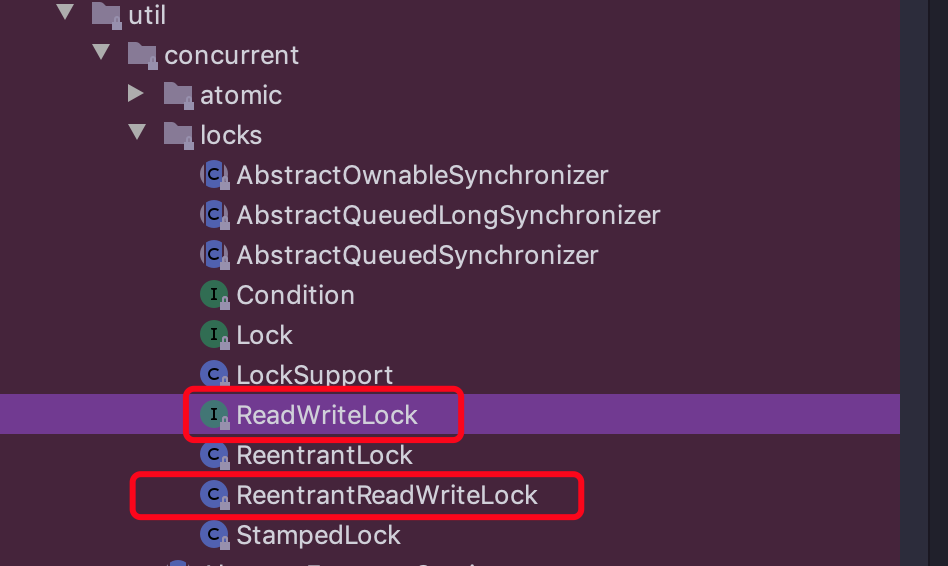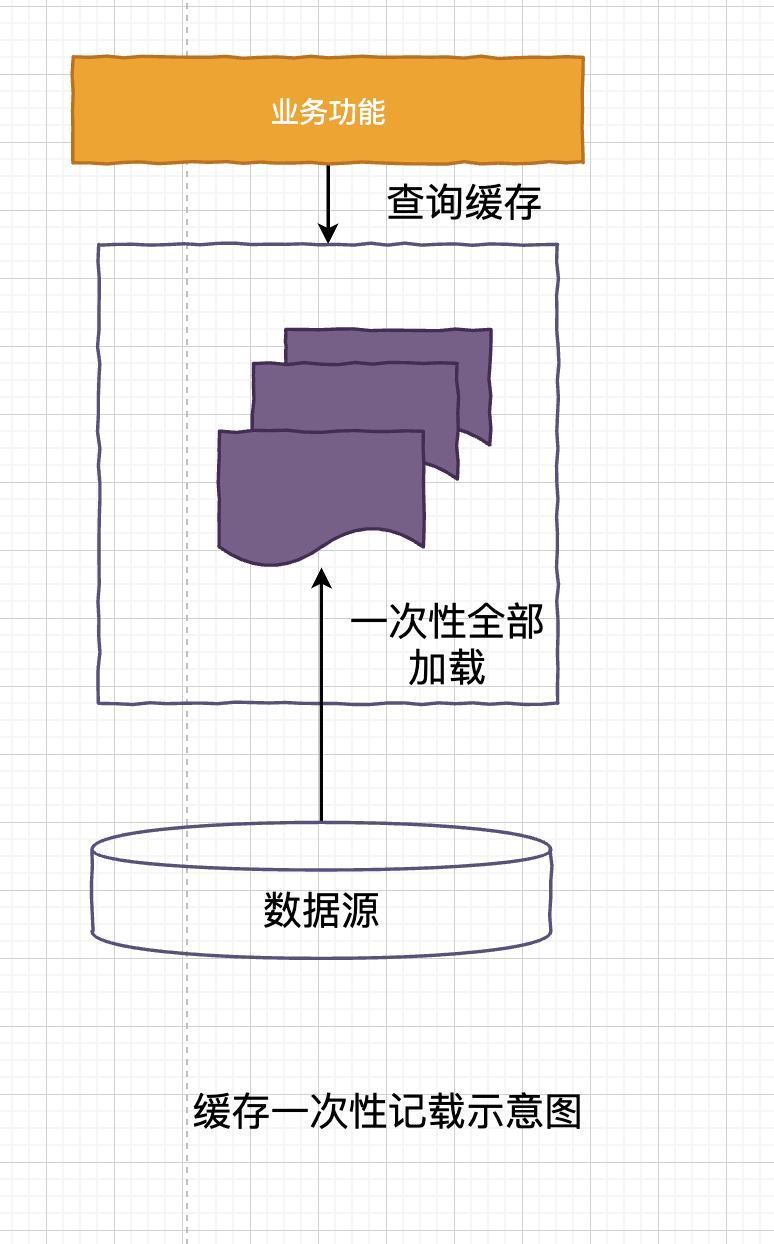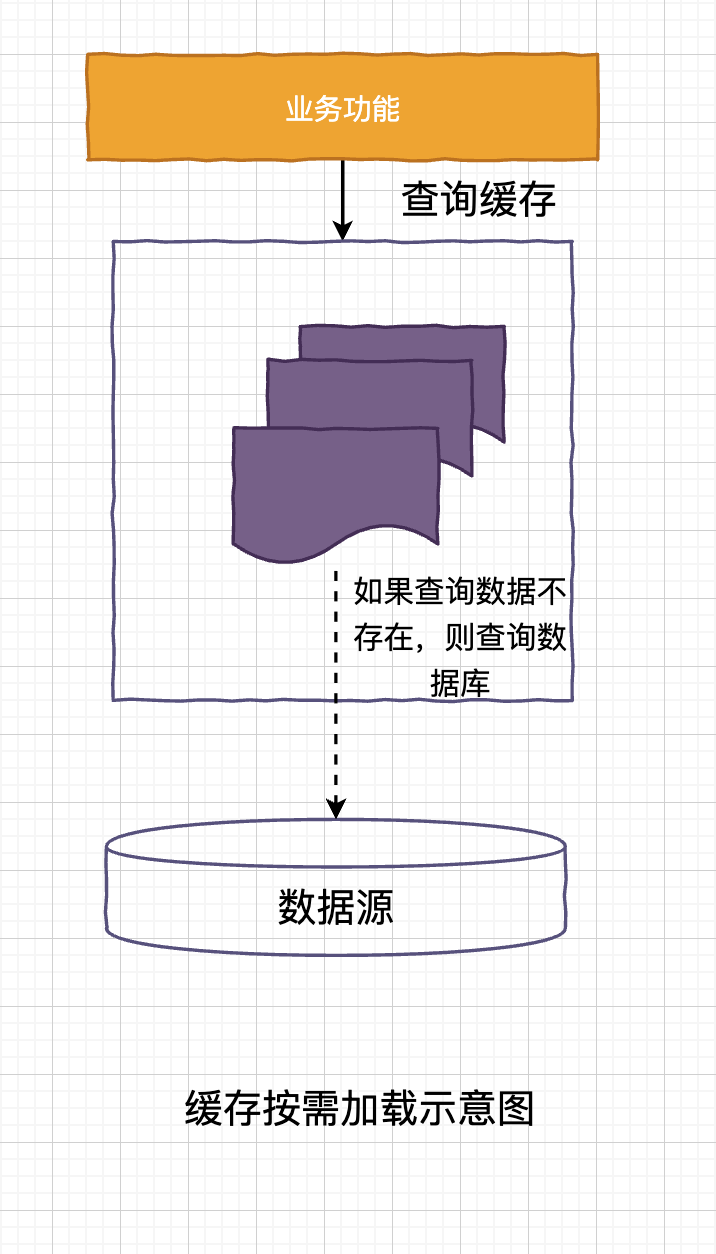# 极客时间 ——《Java并发编程实战》 17 | ReadWriteLock：如何快速实现一个完备的缓存

2020-10-28   15 次阅读

Java 中已经实现了管程信号量这两种同步原语，理论上用这两个任何一个都可以解决所有的并发问题。 但是 JDK 并发包中还存在许多其他的工具类，原因就是：分场景优化性能，提升易用性。### 什么是读写锁

1. 允许多个线程同时读共享变量。
2. 只允许一个线程写共享变量。
3. 如果一个写线程正在执行写操作，此时禁止读线程读共享变量。

• 读写锁允许多个线程同时读共享变量。
• 互斥锁只允许一个线程读/写 共享变量。

### 快速实现一个缓存

ReadWriteLock 是接口，它的具体实现类是 ReentrantReadWriteLock，从名字就可以看出读写锁是支持重入的，下面通过 rwl 创建一把读锁一把写锁

Cache 这个工具类中提供两个方法：

• 读缓存方法：get()
• 写缓存方法 put()

public class Cache<K,V> {
final Map<K, V> m = new HashMap<>();
// 读锁
// 写锁
final Lock w = rwl.writeLock();
// 读缓存
V get(K key) {
r.lock();
try {
return m.get(key);
}finally {
r.unlock();
}
}

// 写缓存
V put(K key, V value) {
w.lock();
try {
return m.put(key, value);
}finally {
w.unlock();
}
}
}### 实现缓存的按需加载

public class Cache<K,V> {
final Map<K, V> m = new HashMap<>();
// 读锁
// 写锁
final Lock w = rwl.writeLock();
V get(K key) {
V v = null;
// 读缓存
r.lock(); // ① 获取读锁
try {
v = m.get(key); // ② 查询缓存
}finally {
r.unlock(); // ③ 释放读锁
}
// 如果缓存中存在对应数据，直接返回
if (v != null) { // ④ 判断缓存数据是否存在
return v;
}
// 缓存中不存在，查询数据库
w.lock();  // ⑤ 获取写锁
try {
// 再次验证数据是否存在，因为数据可能已经被更新
v = m.get(key); // ⑥ 再次验证
if (v == null) { // ⑦
// 查询数据库  v=省略代码
//将查询出的数据放入缓存
m.put(key, v);
}
}finally {
w.unlock();
}
return v;
}
}


### 读写锁的升级与降级


//读缓存
r.lock();         // ① 获取读锁
try {
v = m.get(key); // ②
if (v == null) {
w.lock(); // 获取写锁
try {
//再次验证并更新缓存
//省略详细代码
} finally{
w.unlock();
}
}
} finally{
r.unlock();     // ③
}


public class CachedData {
Object data;
volatile boolean cacheValid;

// 读锁

// 写锁
final Lock w = rwl.writeLock();

void processCachedData() {
// 获取读锁
r.lock();
if (!cacheValid) {
// 释放读锁，因为不允许锁升级
r.unlock();

// 获取写锁
w.lock();
try {
// 再次检查缓存状态
if (!cacheValid) {
//data = ...  获取数据
cacheValid = true;
}
// 释放写锁前，锁降级为读锁
r.lock(); // ①
}finally {
w.unlock();
}
// 此处线程仍然持有读锁
try {
// use(data) 使用数据
}finally {
r.unlock();
}
}
}
}



Q.E.D.